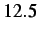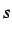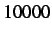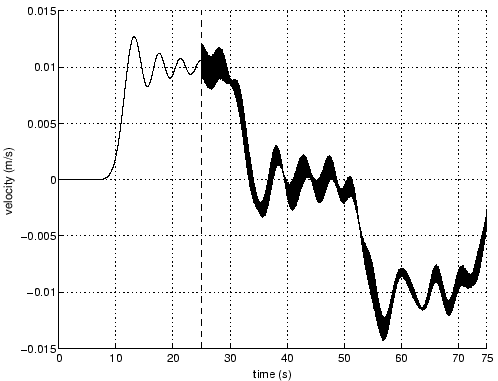Next: Dynamic Insertion with Pre-Stretch Up: Dynamic Cohesive Node Insertion Previous: Blind Cohesive Node Insertion   Contents

Damping of Blind Insertion

Using the reference problem we apply damping during the blind insertion of cohesive nodesthrough. From trial and error we find that the optimal damping parameter, described in Chapter 2, is. Figures 3.15 and 3.16 show the effect that linear damping has on nodeat time steps() and(), respectively. Compared to the velocity profiles due to blind insertion at these same times (Figures 3.13 and 3.14), damping does minimize the previous oscillatory effect. Only minor oscillations are present at the time of insertion, but are then completely dampened out.Although damping does minimize the nodal oscillations, it is quite cumbersome to implement since we use a trial and error approach to determine the optimal damping coefficients. In order to use damping as efficiently as possible, we require an analytical correlation between a given system and the appropriate damping parameters. Unfortunately, derivation of such a correlation is outside the scope of this research and is left for future investigation.Next: Dynamic Insertion with Pre-Stretch Up: Dynamic Cohesive Node Insertion Previous: Blind Cohesive Node Insertion   Contents
Mariusz Zaczek 2002-10-13